# ICSE Worksheet for Chapter - 7 Surds And Exponents Class 8

## Find ICSE Worksheet for chapter-7 Surds And Exponents class 8

1. CLASS-8
2. BOARD: ICSE
3. Mathematic Worksheet - 7
4. TOPIC: Surds And Exponents
5. For otherICSE Worksheet for class 8 Mathematic check out main page of Physics Wallah.

#### SUMMARY:

1. Surds and Radicals:
2. Let ‘a’ be a rational number and ‘n’ be a positive integer such that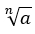is an irrational, thenis called a surd of the order n and a is called the radical.
3.is a surd if a is a rational number andis an irrational number.
4. Law of Radicals:
5.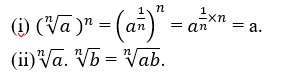6. Exponents:
7. Law of exponents:
8.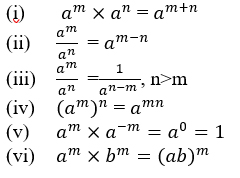#### EXERCISE:

1. If 3x = 500, then the value of 3x-2 is:
1. 100/9
2. 1000/9
3. 500/9
4. 500/3
2. If p/q = (2/3)3 (3/2)3 then the value of (p/q)(-10) is:
1. 1
2. 0
3. cannot be determined
4. None of these
3. The value of [(-2)-2 ]-3 is:
1. 64
2. 32
3. Cannot be determined
4. None of these
4. Simplify: (32)-2/5 (125)-2/3
1. 4/25
2. 25/4
3. 2/5
4. 5/2
5. (64)-2/3×(1/4)-3 equals to:
1. 4
2. 1/4
3. 1
4. 16
6. If x+1/x = 2, then x8+1/x8 is equal to:
1. 1
2. 3
3. 2
4. √2
7. The value of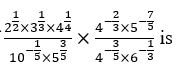is
1. 3/2
2. -3/2
3. 2/3
4. -1/2
8. The exponential form of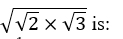1. 6(-1/2)
2. 6(1/2)
3. 6(1/4)
4. 6
9. The value of1. 10
2. 1
3. 6
4. 8
10. Which of the following is equal to 1?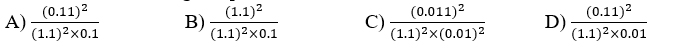11. If apaq=ax, then x=
1. p+q
2. p-q
3. pq
4. p/q
12. The value of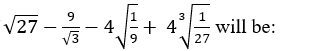1. √3
2. 2√3
3. 0
4. 3√3
13. If (4)3×(6)4×(10)5= 2x×3y×5z then the value of x+y+z is:
1. 12
2. 15
3. 20
4. 24
14. If b=a+c, then (x/y)a (y/x)b (y/x)-c=
1. 0
2. 1
3. 2
4. – 1
15. The value of ‘a’ in the following equation: [9/2]-3×[2/9]-6=[2/9]2a-1 [9/2]0
1. 1
2. - 2
3. - 1
4. 2

1. (C)
2. (A)
3. (A)
4. (B)
5. (A)
6. (C)
7. (B)
8. (C)
9. (A)
10. (C)
11. (A)
12. (C)
13. (D)
14. (B)
15. (C)